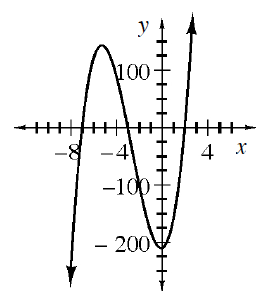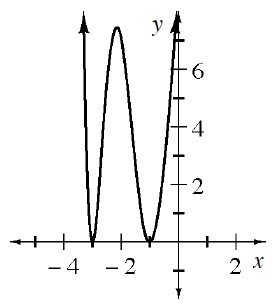### Home > INT3 > Chapter 8 > Lesson 8.1.2 > Problem8-34

8-34.

What is the degree of each polynomial function below? For parts (c) and (d), state the minimum degree.

1. $P\left(x\right) = 0.08x^{2} + 28x$

Look for the highest-valued exponent.

$2$

1. $y=8x^2-\frac{1}{7}x^5+9$

See part (a).

1.If you shifted the graph vertically, what is the maximum number of $x$-intercepts it could have?

1.See part (c).

$4$